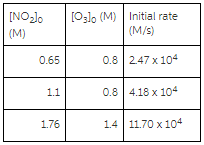Problem: Consider this initial-rate data at a certain temperature for the reaction described by 2NO2(g) + O3(g) → N2O5 (g) +O2 (g) Determine the value and units of the rate constant.

FREE Expert Solution
94% (344 ratings)
Problem Details

Consider this initial-rate data at a certain temperature for the reaction described by

2NO2(g) + O3(g) → N2O5 (g) +O2 (g)

Determine the value and units of the rate constant.What scientific concept do you need to know in order to solve this problem?

Our tutors have indicated that to solve this problem you will need to apply the Rate Law concept. You can view video lessons to learn Rate Law Or if you need more Rate Law practice, you can also practice Rate Law practice problems .

What is the difficulty of this problem?

Our tutors rated the difficulty of Consider this initial-rate data at a certain temperature for... as high difficulty.

How long does this problem take to solve?

Our expert Chemistry tutor, Dasha took 9 minutes to solve this problem. You can follow their steps in the video explanation above.

What professor is this problem relevant for?

Based on our data, we think this problem is relevant for Professor Laskin & Skrynnikov's class at PURDUE.